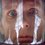# Playing with Integrals: Ineqaulitites and Integrals 2

Although my previous post on this topic didn't cause a discussion I'll try to continue. If no one will be interested in problems from this post too, I'll switch to another theme.

Today I would like to present several inequalities directly involving integrals.

Problem 4. If $f:[0,1]\rightarrow[0,1]$ is a continuous function, prove the following inequality $\left(\int^1_0f(x)\,dx\right)^2\leq 2\int^1_0xf(x)\,dx$

Solution. To start our proof we'll consider the following function $F(t)=\left(\int^t_0f(x)\,dx\right)^2- 2\int^t_0xf(x)\,dx$ We know that $F(0)=0$, so to prove our inequality we simply need to prove taht $F(t)$ is a decreasing fucntion. $F^\prime(t)=2f(t)\int^t_0f(x)\,dx-2tf(t)=2f(t)\int^1_0(f(x)-1)\,dx$ However, we know that range of $f(x)$ is $[0,1]$, so $f(x)-1\leq 0$, subsequently by Theorem 1. $\displaystyle\int^1_0(f(x)-1)\,dx\leq 0$. So $F^\prime(t)=2f(t)\int^1_0(f(x)-1)\,dx\leq 0,$ which means that $F(t)$ is decreasing and $F(1)\leq F(0)=0$. Finaly we arrvie at the desired result $\left(\int^1_0f(x)\,dx\right)^2\leq 2\int^1_0xf(x)\,dx.$

Now try your own techniques to solve the following problems.

Problem 5. For continuous, differentiable function $f:[0,1]\rightarrow\mathbb{R}$, there exists $a\in(0,1)$ such that $\displaystyle\int^a_0f(x)\,dx=0$. Prove that

$\left|\int^1_0f(x)\,dx\right|\leq\frac{1-a}{2}\sup_{x\in(0,1)}|f^\prime(x)|$

Hint. For this problem you might consider the Mean Value Theorem

Problem 6. Let $f:[0,1]\rightarrow\mathbb{R}$ be a continuous function satisfying following property $\int^1_0 f(x)\,dx=\int^1_0 xf(x)\,dx=1$ Prove that $\int^1_0 f^2(x)\,dx\geq 4$

Hint. Try to search for a linear fucntion with the same proprties, also here $f^2(x)=f(x)\cdot f(x)$.Note by Nicolae Sapoval
7 years, 6 months ago

This discussion board is a place to discuss our Daily Challenges and the math and science related to those challenges. Explanations are more than just a solution — they should explain the steps and thinking strategies that you used to obtain the solution. Comments should further the discussion of math and science.

When posting on Brilliant:

• Use the emojis to react to an explanation, whether you're congratulating a job well done , or just really confused .
• Ask specific questions about the challenge or the steps in somebody's explanation. Well-posed questions can add a lot to the discussion, but posting "I don't understand!" doesn't help anyone.
• Try to contribute something new to the discussion, whether it is an extension, generalization or other idea related to the challenge.

MarkdownAppears as
*italics* or _italics_ italics
**bold** or __bold__ bold
- bulleted- list
• bulleted
• list
1. numbered2. list
1. numbered
2. list
Note: you must add a full line of space before and after lists for them to show up correctly
paragraph 1paragraph 2

paragraph 1

paragraph 2

[example link](https://brilliant.org)example link
> This is a quote
This is a quote
    # I indented these lines
# 4 spaces, and now they show
# up as a code block.

print "hello world"
# I indented these lines
# 4 spaces, and now they show
# up as a code block.

print "hello world"
MathAppears as
Remember to wrap math in $$ ... $$ or $ ... $ to ensure proper formatting.
2 \times 3 $2 \times 3$
2^{34} $2^{34}$
a_{i-1} $a_{i-1}$
\frac{2}{3} $\frac{2}{3}$
\sqrt{2} $\sqrt{2}$
\sum_{i=1}^3 $\sum_{i=1}^3$
\sin \theta $\sin \theta$
\boxed{123} $\boxed{123}$

Sort by:

Please do continue. I like your posts very much and I am always a fan of inequalities and a newcommer at Calculus.Please do such another ones.Thanks.

- 7 years, 6 months ago

In problem 4, I think the statement should be $\left( \int_0^1 f(x) \: dx \right)^2 \le 2 \int_0^1 xf(x) \: dx.$ This is the only way the expression for $F'(t)$ makes sense. (Also, there exist counter-examples to the statement as is.)

- 7 years, 6 months ago

Yes, you're definitely right. Thanks for pointing that out!

- 7 years, 6 months ago